# Spiral

example processing for-loop pretty 100-days-of-code
``````size(500, 500);

strokeWeight(4);
background(32);

float distance = 0;

float r = random(256);
float g = random(256);
float b = random(256);
float cChange = 2;
float distanceChange = .001;

for (float angle = 0; angle < 360*100; angle += .1) {

float x = width/2 + cos(radians(angle))*distance;
float y = height/2 + sin(radians(angle))*distance;

r += random(-cChange, cChange);
r = constrain(r, 0, 256);

g += random(-cChange, cChange);
g = constrain(g, 0, 256);

b += random(-cChange, cChange);
b = constrain(b, 0, 256);

stroke(r, g, b);

point(x, y);

distance += distanceChange;
}
``````
Code Editor

See the Pen by Happy Coding (@KevinWorkman) on CodePen.

This code uses a `for` loop to go around a circle 100 times, incrementing by `.1` degrees. In other words, it takes 360,000 steps to go around the cirlce 100 times! (That means it might take a little bit until you see anything when you run the code.)

Each iteration of the loop, the code uses the `cos()` and `sin()` functions to figure out where in the spiral it is. It then chooses a color and draws a point, and then increases the distance before it repeats. Increasing the distance causes the point to spiral out from the center.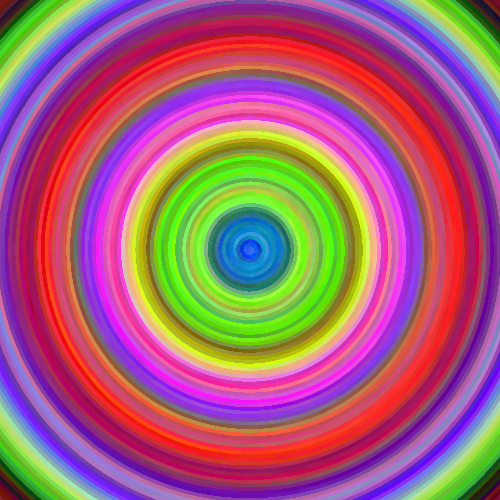You can play with how much the distance increases, or the start point, or the colors to create different effects.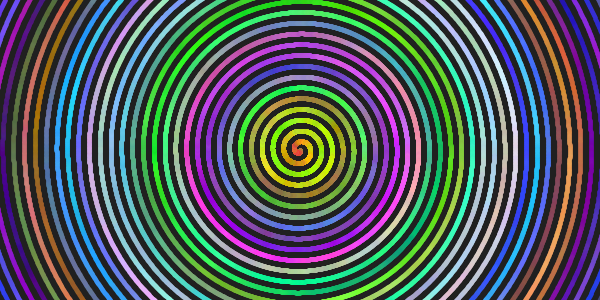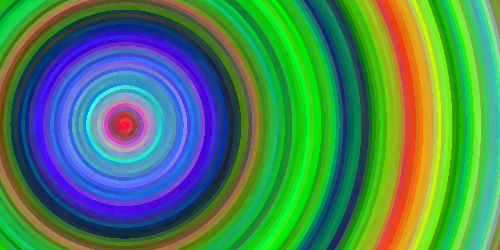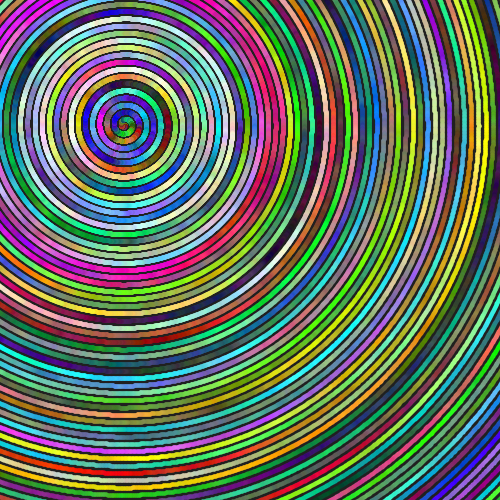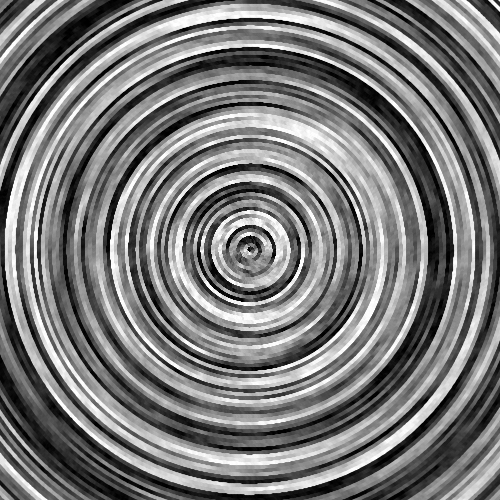## Tweak Ideas

• Change how much the distance increases. What happens if you make this value larger or smaller?
• Change the start point, or make multiple spirals.
• Change the colors so the spiral is black and white, or a shade of blue. Make it so the spiral gets darker or lighter as it gets further away.
• Make it so the spiral randomly decreases in distance instead of only ever increasing.